# 强化学习笔记（2）——马尔可夫决策过程

## 1. 马尔可夫过程（Markov Process，MP）

1. 如果一个状态转移是符合马尔可夫的，那就是说一个状态的下一个状态只取决于它当前状态，而跟它当前状态之前的状态都没有关系。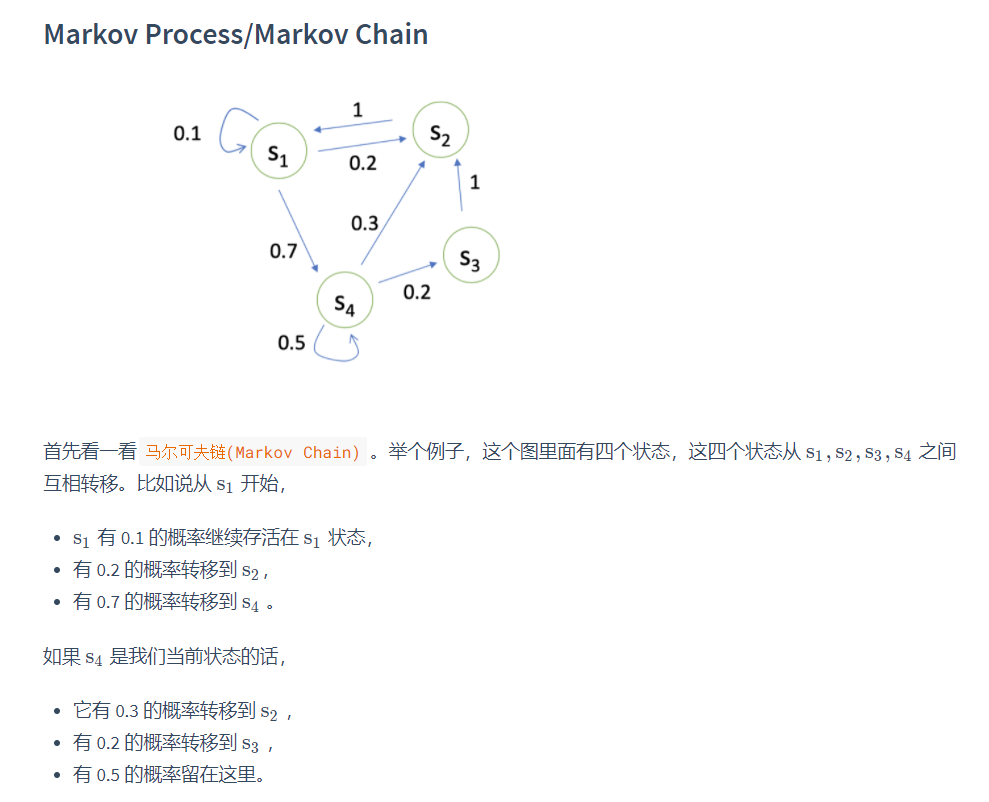我们可以用状态转移矩阵(State Transition Matrix) P 来描述状态转移 p ( s t + 1 = s ′ ∣ s t = s ) p\left(s_{t+1}=s^{\prime} \mid s_{t}=s\right) ，如下式所示。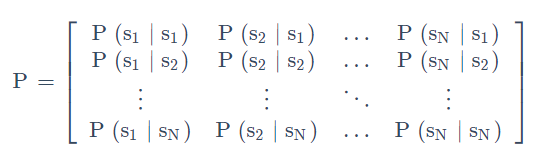## 2. 马尔可夫奖励过程（Markov Reward Process，MRP）

马尔可夫奖励过程(Markov Reward Process, MRP) 是马尔可夫链再加上了一个奖励函数。

1. Horizon是指一个回合的长度（每个回合最大的时间步数），它是由有限个步数决定的。

2. Return(回报) 说的是把奖励进行折扣后所获得的收益。Return 可以定义为奖励的逐步叠加，如下式所示：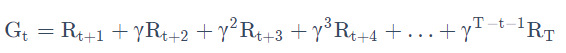其中：

• R t + 1 R_{t+1} 代表从状态 s t s_t 转移到 s t + 1 s_{t+1} 得到的奖励
• G t G_t 代表在状态 s t s_t 下能获得的折扣回报
这里有一个叠加系数，越往后得到的奖励，折扣得越多。这说明我们其实更希望得到现有的奖励，未来的奖励就要把它打折扣。
1. 当我们有了 return 过后，就可以定义一个状态的价值了，就是state value function。对于 MRP，state value function 被定义成是 return 的期望，如下式所示：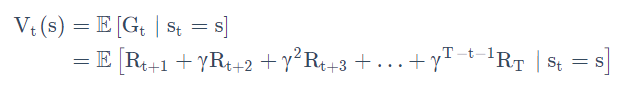G t G_t 是之前定义的 discounted return，我们这里取了一个期望，期望就是说从这个状态开始，你有可能获得多大的价值。discount factor 可以作为强化学习 agent 的一个超参数来进行调整，然后就会得到不同行为的 agent。

### 2.1 迭代法计算状态价值函数 V V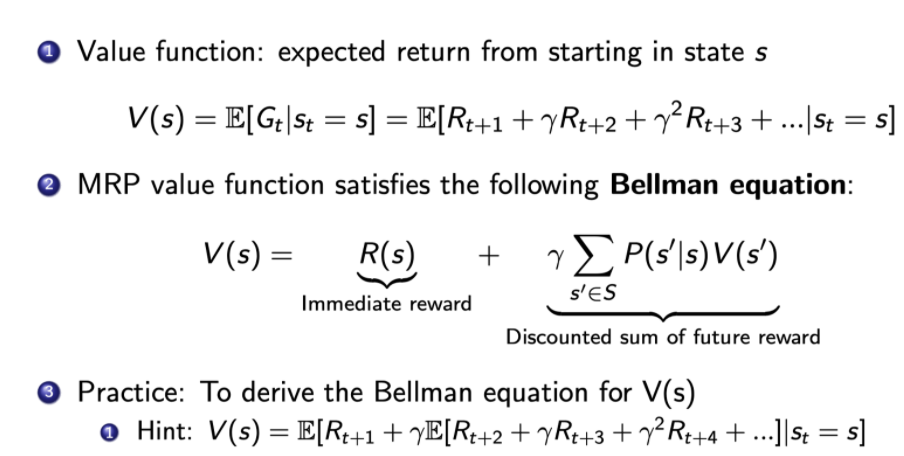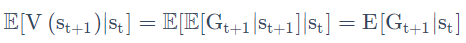• V ( s t + 1 ) V(s_{t+1}) 代表在状态 s t + 1 s_{t+1} 下的状态价值函数
• G t + 1 G_{t+1} 代表在状态 s t + 1 s_{t+1} 下的折扣回报（discounted return）。从 s t + 1 s_{t+1} 转移到 s t + 2 s_{t+2} 的奖励 R t + 2 R_{t+2} 开始算起，后面乘以折扣因子再相加。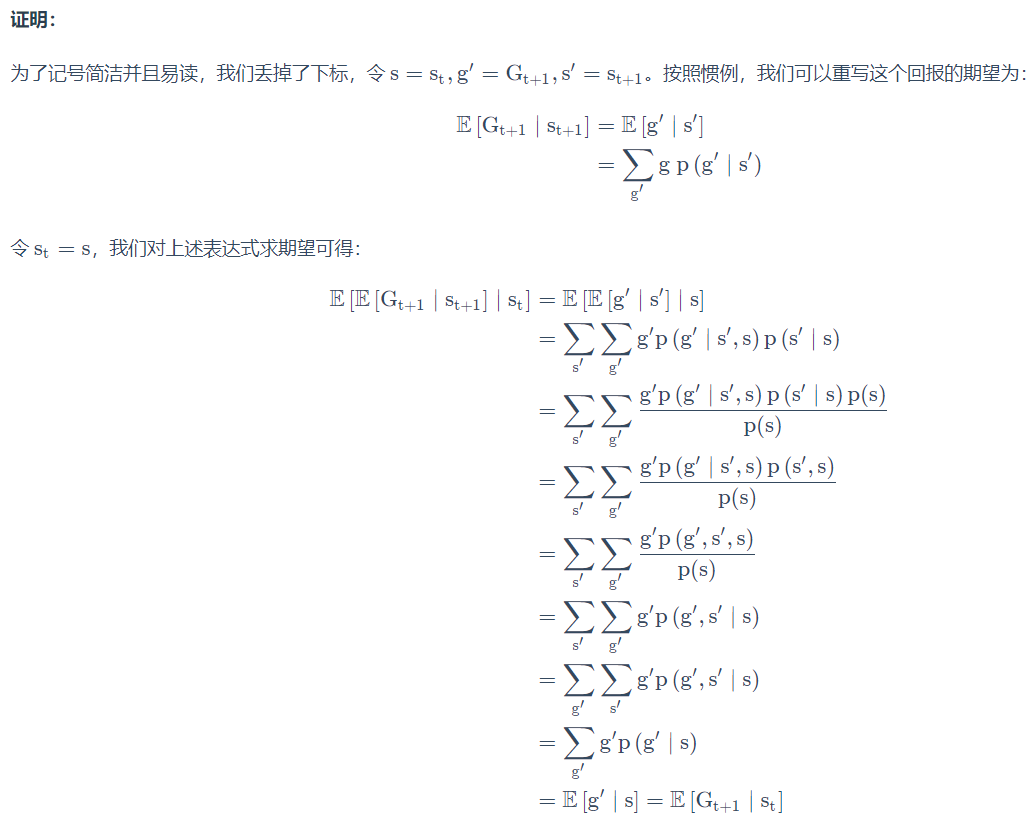如何理解全期望公式？表面上看，好像 G t + 1 G_{t+1} V ( s t + 1 ) V(s_{t+1}) 计算更简洁，计算得到了优化，实际上，在计算 E [ G t + 1 ∣ s t ] \mathrm{E}\left[\mathrm{G}_{\mathrm{t}+1} \mid \mathrm{s}_{\mathrm{t}}\right] 的时候仍然要考虑状态 s t s_{t} 转移到状态 s t + 1 s_{t+1} 的概率和状态 s t + 1 s_{t+1} 转移到状态 s t + 2 s_{t+2} 的概率，因为 G t + 1 G_{t+1} 并不是状态 s t + 1 s_{t+1} 一个确定的折扣奖励，而是一个随机变量。全期望公式只是形式上得到了简化，但是实际在计算的时候，还是没有得到简化。

贝尔曼等式：定义了当前状态价值函数与未来状态价值函数的关系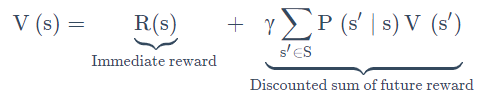• s’可以看成未来的所有状态。
• 转移 P(s’|s)是指从当前状态转移到未来状态的概率。
• V(s’)代表的是未来某一个状态的价值。我们从当前这个位置开始，有一定的概率去到未来的所有状态，所以我们要把这个概率也写上去，这个转移矩阵也写上去，然后我们就得到了未来状态，然后再乘以一个 γ，这样就可以把未来的奖励打折扣。
• 第二部分可以看成是未来奖励的折扣总和(Discounted sum of future reward)。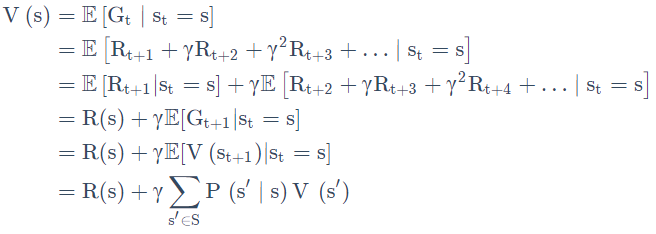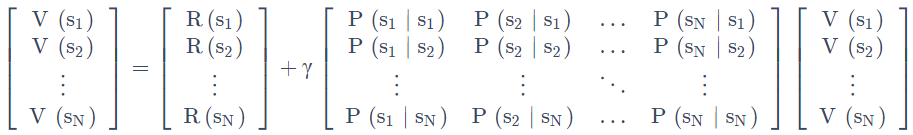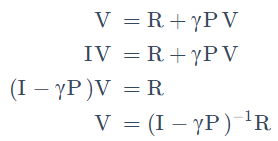### 2.2 蒙特卡罗法计算状态价值函数 V V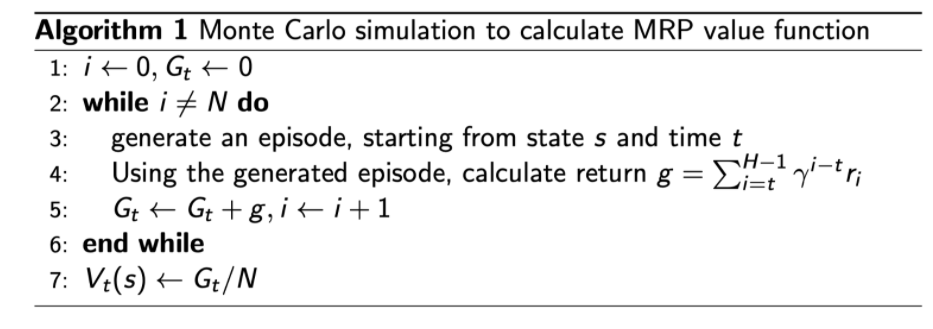### 2.3 动态规划法计算状态价值函数 V V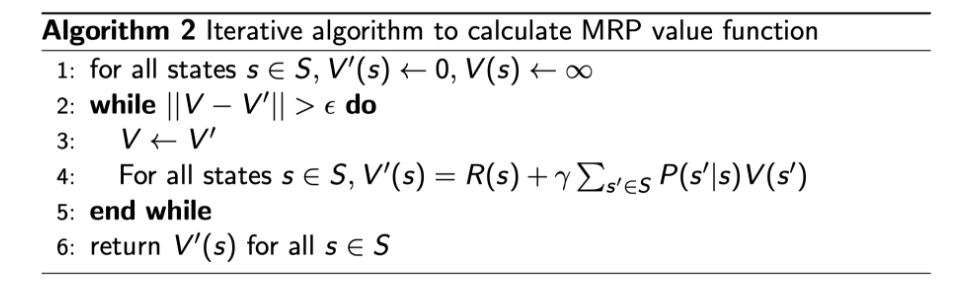## 3. 马尔可夫决策过程（Markov Decision Process，MDP）

• 这里多了一个决策，多了一个动作。
• 状态转移也多了一个条件，变成了 P ( s t + 1 = s ′ ∣ s t = s , a t = a ) P\left(s_{t+1}=s^{\prime} \mid s_{t}=s, a_{t}=a\right) 。未来的状态不仅是依赖于你当前的状态 s s ，也依赖于在当前状态 agent 采取的这个动作 a a
• 对于这个价值函数，它也是多了一个条件，多了一个你当前的动作，变成了 R ( s t = s , a t = a ) = E [ r t ∣ s t = s , a t = a ] R\left(s_{t}=s, a_{t}=a\right)=\mathbb{E}\left[r_{t} \mid s_{t}=s, a_{t}=a\right] 。你当前的状态以及你采取的动作会决定你在当前可能得到的奖励多少。

### 3.1 MDP中的策略policy

• Policy 定义了在某一个状态应该采取什么样的动作。
• 知道当前状态过后，我们可以把当前状态带入 policy function，然后就会得到一个概率，即：
π ( a ∣ s ) = P ( a t = a ∣ s t = s ) \pi(a \mid s)=P\left(a_{t}=a \mid s_{t}=s\right)
假设这个概率函数应该是稳定的(stationary)
• 另外这个策略也可能是确定的，它有可能是直接输出一个值，告诉你当前应该采取什么样的动作，而不是一个动作的概率。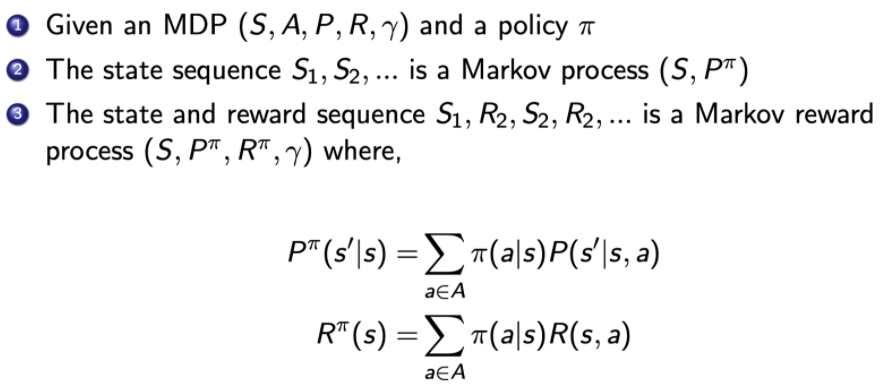### 3.2 MDP和MRP之间的区别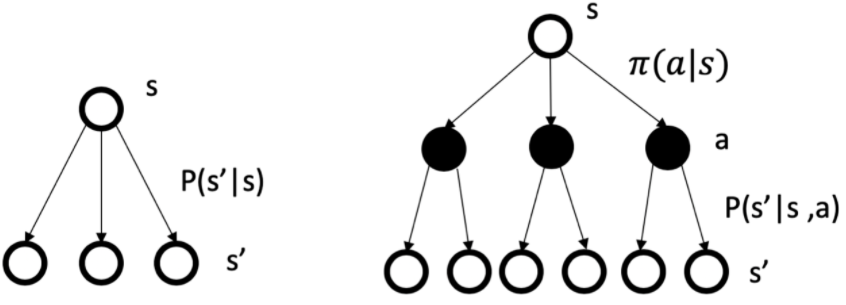### 3.3 MDP的价值函数

1. 仿照MRP中的状态价值函数的定义，可以类似地定义MDP中的价值函数：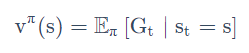我们通过对policy 进行采样来得到一个期望，那么就可以计算出它的价值函数。

2. 这里我们另外引入了一个 Q 函数(Q-function)Q 函数也被称为 action-value function。Q 函数定义的是在某一个状态采取某一个动作，它有可能得到的这个 return 的一个期望。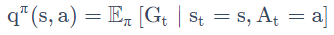对 Q 函数 q π ( s , a ) \rm q^{\pi}(s,a) 中的动作进行加和，就可以得到价值函数 v π ( s ) \rm v^{\pi}(s) ，即：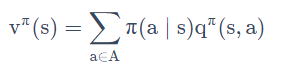3. Q 函数的 Bellman equation：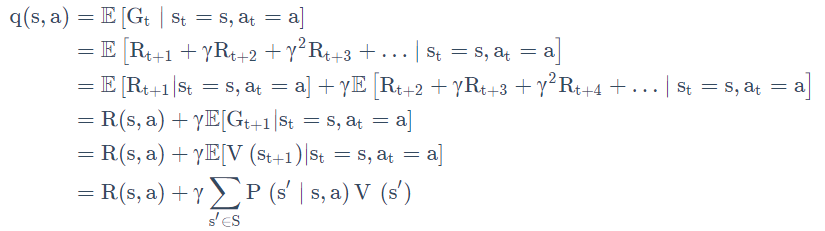### 3.4 贝尔曼期望等式（Bellman Expectation Equation）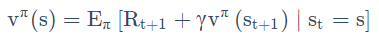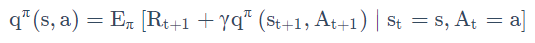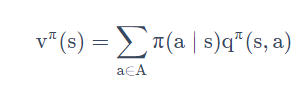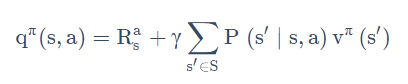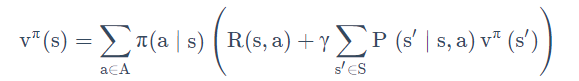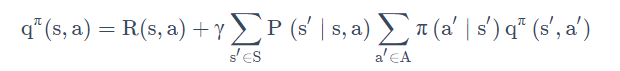### 3.5 备份图（backup diagram）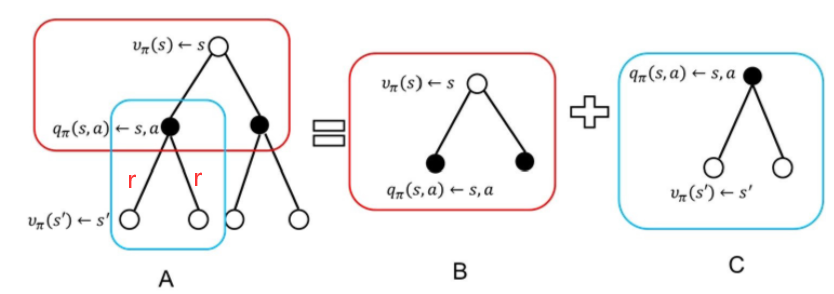• 这些操作将价值信息从一个状态（或状态-动作对）的后继状态（或状态-动作对）转移回它。
• 每一个空心圆圈代表一个状态，每一个实心圆圈代表一个状态-动作对。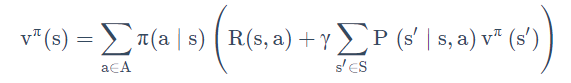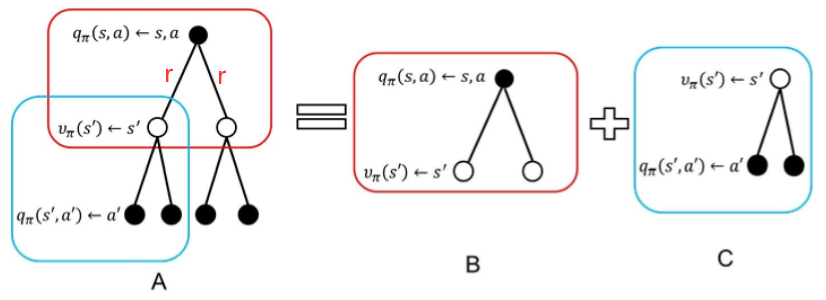• 第一层加和是先把这个叶子节点从黑色节点推到这个白色的节点，进了它的这个状态。
• 当我们到达某一个状态过后，再对这个白色节点进行一个加和，这样就把它重新推回到当前时刻的一个 Q 函数。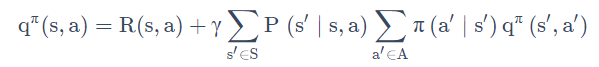### 3.6 预测和控制问题

MDP 的 prediction 和 control 是 MDP 里面的核心问题。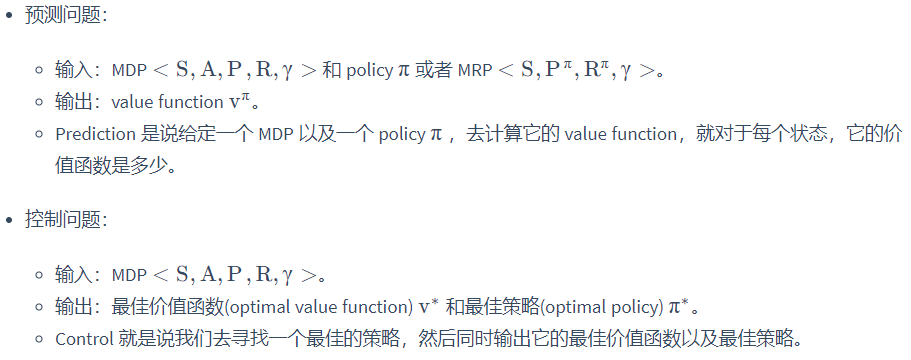#### 3.6.1 解决预测问题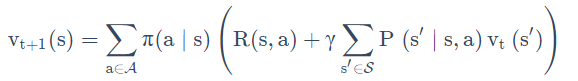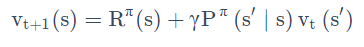#### 3.6.2 解决控制问题

1. 首先来看policy iteration。因为策略 π \pi 和价值函数 v ( s ) \rm v(s) 都不知道，所以需要先假定一个策略，然后按这个策略计算价值函数和Q函数，接着按照使Q函数取得最大值的动作（其他动作被选取到的概率为0）制定新策略，然后再计算价值函数和Q函数，如此往复，直至价值函数和策略收敛。
收敛后，就得到如下等式：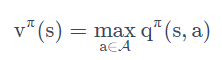上式被称为Bellman optimality equation，它表达了这样一个事实：最佳策略下的一个状态的价值必须等于在这个状态下采取最好动作得到的回报期望。

2. 再来看value iteration。同样是策略 π \pi 和价值函数 v ( s ) \rm v(s) 都不知道，但是value iteration的思路是先按照当前最优动作去迭代价值函数 v ( s ) \rm v(s) ，直到价值函数 v ( s ) \rm v(s) 收敛后，再来制定策略。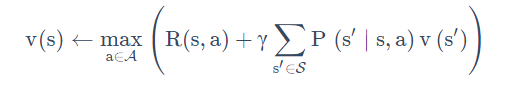07-1723
10-17
03-292915
05-01365
10-122038
10-26
04-20
07-03486
10-12672
05-046895
12-271万+
11-243万+
05-09684
10-23318
07-181万+

### “相关推荐”对你有帮助么？

•非常没帮助
•没帮助
•一般
•有帮助
•非常有帮助被折叠的  条评论 为什么被折叠?到【灌水乐园】发言ReEchooo

¥1 ¥2 ¥4 ¥6 ¥10 ¥20获取中扫码支付点击重新获取扫码支付1.余额是钱包充值的虚拟货币，按照1:1的比例进行支付金额的抵扣。
2.余额无法直接购买下载，可以购买VIP、付费专栏及课程。余额充值